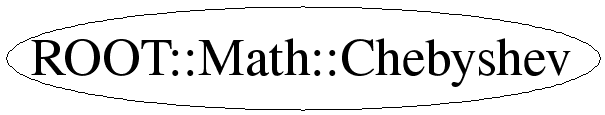# class ROOT::Math::Chebyshev

```
Class describing a Chebyshev series which can be used to approximate a
function in a defined range [a,b] using Chebyshev polynomials.
It uses the algorithm from
<A HREF="http://www.gnu.org/software/gsl/manual/html_node/Chebyshev-Approximations.html">GSL</A>

This class does not support copying
@ingroup FuncApprox

```

## Function Members (Methods)

public:
 virtual ~Chebyshev() ROOT::Math::Chebyshev Chebyshev(const ROOT::Math::IGenFunction& f, double a, double b, size_t n) ROOT::Math::Chebyshev Chebyshev(ROOT::Math::GSLFuncPointer f, void* p, double a, double b, size_t n) ROOT::Math::Chebyshev* Deriv() pair EvalErr(double x) const pair EvalErr(double x, size_t n) const ROOT::Math::Chebyshev* Integral() double operator()(double x) const double operator()(double x, size_t n) const
protected:
 void Initialize(ROOT::Math::GSLFuncPointer f, void* params, double a, double b)
private:
 ROOT::Math::Chebyshev Chebyshev(size_t n) ROOT::Math::Chebyshev Chebyshev(const ROOT::Math::Chebyshev&) ROOT::Math::Chebyshev& operator=(const ROOT::Math::Chebyshev&)

## Data Members

private:
 ROOT::Math::GSLFunctionWrapper* fFunction pointer to function size_t fOrder ROOT::Math::GSLChebSeries* fSeries

## Class Charts## Function documentation

Chebyshev(const ROOT::Math::IGenFunction& f, double a, double b, size_t n)
```Construct a Chebyshev series approximation to a Function f in range [a,b];
constructor based on functions of type IGenFunction

```
Chebyshev(ROOT::Math::GSLFuncPointer f, void* p, double a, double b, size_t n)
```Construct a Chebyshev series approximation to a Function f in range [a,b];
constructor based on free functions with gsl_function type signature

```
virtual ~Chebyshev()
``` destructor
```

```construct a Chebyshev series or order n
The series must be initialized from a function

```
Chebyshev(const Chebyshev &)
``` usually copying is non trivial, so we make this unaccessible
```
double operator()(double x) const
```Evaluate the series at a given point x

```
std::pair<double, double> EvalErr(double x) const
```Evaluate the series at a given point x estimating both the series result and its absolute error.
The error estimate is made from the first neglected term in the series.
A pair containing result and error is returned

```
double operator()(double x, size_t n) const
```Evaluate the series at a given point, to (at most) the given order n

```
std::pair<double, double> EvalErr(double x, size_t n) const
```evaluate the series at a given point x to the given order n,
estimating both the series result and its absolute error.
The error estimate is made from the first neglected term in the series.
A pair containing result and error is returned

```

```Compute the derivative of the series and return a pointer to a new Chebyshev series with the
derivatives coefficients. The returned pointer must be managed by the user.

TO DO: implement copying to return by value
```

```Compute the integral of the series and return a pointer to a new Chebyshev series with the
integral coefficients. The lower limit of the integration is the left range value a.
The returned pointer must be managed by the user

TO DO: implement copying to return by value
```
void Initialize(ROOT::Math::GSLFuncPointer f, void* params, double a, double b)
```Initialize series passing function and range

```

Last update: root/mathmore:\$Id: Chebyshev.h 21503 2007-12-19 17:34:54Z moneta \$
Copyright (c) 2004 ROOT Foundation, CERN/PH-SFT *

This page has been automatically generated. If you have any comments or suggestions about the page layout send a mail to ROOT support, or contact the developers with any questions or problems regarding ROOT.